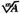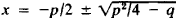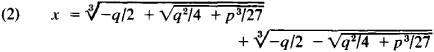# Galois Theory

Also found in: Dictionary, Thesaurus, Wikipedia.

## Galois theory

[′gal‚wä ‚thē·ə·rē]
(mathematics)
The study of the Galois field and Galois group corresponding to a polynomial.
McGraw-Hill Dictionary of Scientific & Technical Terms, 6E, Copyright © 2003 by The McGraw-Hill Companies, Inc.
The following article is from The Great Soviet Encyclopedia (1979). It might be outdated or ideologically biased.

## Galois Theory

the theory created by E. Galois of higher degree algebraic equations with one unknown, that is, of equations having the form

(1) xn + a1xn-1 + a2xn-2 + … + + an-1x + an = 0

The theory determines the conditions for reducing the solution of such equations to the solution of a chain of other algebraic equations (usually of lower degree). Inasmuch as the solution of the binomial equation xm = A is the radical, equation (1) is solvable by radicals if it can be reduced to a chain of binomial equations. All equations of degrees two, three, and four are solvable by radicals. The second-degree equation x2 + px + q = 0 was solved in very ancient times by the well-known formula. Third- and fourth-degree equations were solved in the 16th century. For third-degree equations having the form x3 + px + q = 0 (to which all third-degree equations can be reduced), a solution is given by the so-called Cardan’s formulaIt was published by J. Cardan in 1545, although the question of whether he discovered it himself or borrowed it from other mathematicians cannot be considered entirely resolved. The method of solving fourth-degree equations by radicals was found by L. Ferrari.

In the course of the next three centuries, mathematicians attempted to find similar formulas for equations of degree five or higher. E. Bezout and J. Lagrange worked persistently on this problem. The latter examined special linear combinations of the roots (the so-called Lagrange resolvents) as well as the question of which equations are satisfied by the rational functions of the roots of equation (1). In 1801, K. Gauss developed a complete theory of a solution by radicals for a binomial equation of the form xn = 1, in which he reduced the solution of such an equation to the solution of a chain of binomial equations of lower degrees and gave the necessary and sufficient conditions for solving the equation xn = 1 in square roots. From a geometric viewpoint, the latter problem consists in finding n-sided regular polygons that can be drawn with a compass and a ruler; consequently the equation xn = 1 is called the cyclotomic equation. Finally, N. Abel demonstrated in 1824 that the general fifth-degree equation (and especially general equations of higher degrees) are not solvable by radicals. On the other hand, Abel gave a solution by radicals for one general class of equations that includes equations of arbitrarily high degrees, the so-called Abelian equations.

Thus, when Galois began his studies much had been accomplished in the theory of algebraic equations but a general theory covering all possible equations of the form of equation (1) had not yet been developed. For instance, it remained (1) to establish the necessary and sufficient conditions that equation (1) must satisfy so that it would be solvable by radicals, (2) to find the chain of simpler equations, even if they were not binomial equations, to which the solution of the given equation (1) could be reduced, and especially (3) to ascertain the necessary and sufficient conditions such that the equation (1) could be reduced to a chain of quadratic equations (that is, so that the roots of the equation could be constructed geometrically with a compass and ruler). Galois answered all these questions in his Memoir on the Conditions of Solvability of Equations by Radicals, which was found among his papers after his death and was first published by J. Liouville in 1846. In order to answer these questions, Galois studied the profound relationships between the properties of equations and groups of substitutions, introducing a number of fundamental concepts of group theory. He formulated his condition of solvability of equation (1) by radicals using group theory terminology. After Galois, his theory was expanded and generalized in many directions. In the modern conception, it is a theory that examines various mathematical entities on the basis of their groups of automorphisms (thus, for example, it is possible to have a Galois theory of fields, of rings, of topological spaces, and so forth).

### REFERENCES

Galois, E. Sochineniia. Moscow-Leningrad, 1936. (Translated from French.)
Chebotarev, N. G. Osnovy teorii Galua, vols. 1-2. Moscow-Leningrad, 1934-37.
Postnikov, M. M. Teoriia Galua. Moscow, 1963.
References in periodicals archive ?
Ramis, "Integrability of dynamical systems through differential Galois theory: a practical guide," in Differential Algebra, Complex Analysis and Orthogonal Polynomials, P.
The most remote sources of partial actions of Hopf algebras can be found in the theory of partial Galois extensions, which was a generalization of the Galois theory for commutative rings by Chase, Harrison and Rosenberg to the case of partial group actions.
In Section 2 we recall some basic notions of categorical Galois theory and we prove our criterion for reflectiveness of normal extensions.
Let us begin with what is usually called Grothendieck's formulation of the fundamental theorem of Galois theory:
Swan, Noether's problem in Galois theory, in Emmy Noether in Bryn Mawr (Bryn Mawr, Pa., 1982), edited by B.
Other topics include polynomials, factorization in integral domains, p-groups and the Sylow theorems, Galois theory, and finiteness conditions for rings and modules.
He begins with a discussion of Lie group theory's intellectual underpinnings in Galois theory and concludes with a chapter on the application of Lie group theory to solving differential equations, both subjects that are relatively rare in texts on Lie group theory.
Pollack introduces algebraic number theory to readers who are familiar with linear algebra, commutative ring theory, Galois theory, a little abelian groups theory, and elementary number theory up to and including the law of quadratic reciprocity.
Since Gal(K/Q) = [D.sub.8] contains three distinct subgroups of index 2, there are three distinct quadratic subextensions in K/Q by Galois theory. Since all prime numbers ramified in K/Q are only [p.sub.1] and [p.sub.2], these three quadratic extensions must be [k.sub.1], [k.sub.2] and Q([square root of [p.sub.1][[p.sub.2]]).
The papers discuss recent developments in the field and describe several approaches to the geometrical and algebraic aspects of differential and difference equations, such as Lie symmetry groups and their invariants, differential Galois theory, group theoretical methods in physics, and geometrization of mechanics.
Serre focuses primarily on the inverse problem of Galois theory, that being the construction of field extensions having a given finite groups as Galois groups.
After introducing notation and Zorn's lemma, he arranges the problems in chapters on integers and integers mod &lt;n/&gt;, groups, rings, linear algebra and canonical forms of linear transformations, and fields and Galois theory. ([umlaut] Ringgold, Inc., Portland, OR)

Site: Follow: Share:
Open / Close